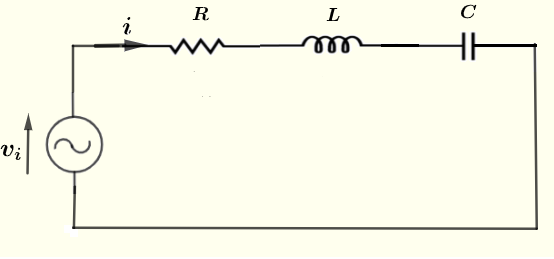# RLC Series Current Graphing Calculator

 

An online graphing calculator of the RLC series circuit current as a function the angular frequency $\omega$ is presented.
This calculator graphs the amplitude $| I |$ and phase $P$ as a function of the angular frequecy $\omega = 2 \pi f$ and calculates the frequency of resonnance $\omega_r$, the lower and higher cutoff frequencies $\omega_L$, $\omega_H$, the quality factor $Q$ and the bandwidth $\Delta \omega$ of the RLC series circuit.

## RLC Series Circuit

It has been shown that the current $I$ in a series RLC circuit shown below.is given by:
$I = \dfrac{V_0}{ \sqrt {R^2 + \left(\omega L - \dfrac{1}{\omega C} \right)^2} }$
where $V_0$ is the peak value of the voltage source $v_i = V_0 \cos (\omega t)$.
The frequency of resonance is defined as the frequency for which $I$ is maximum and is given by.
$\omega_r = \dfrac{1}{\sqrt {L C}} \quad \quad (I)$
This calculator calculate the amplitude $|I|$ of the current $I$ and its phase $P$ defined as
$|I| = \sqrt {I_r^2 + I_i^2}$ $P = \arctan 2 (I_i , I_r)$ where $I_r$ be the real part of $I$ and let $I_i$ be the imagianry part of $I$.
The cutoff frequencies are:
$\omega_L = \dfrac {- R C + \sqrt{ (R C)^2 + 4 L C }}{ 2 L C }$

$\omega_H = \dfrac {R C + \sqrt{ (R C)^2 + 4 L C}}{ 2 L C }$
The bandwidth of the resonant circuit is defined by: $\Delta \omega = \omega_H - \omega_L$
The quality factor $Q$ is given by
$Q = \omega_r \dfrac{L}{R} \quad \quad$
This calculator graphs the amplitude $| I |$ and phase $P$ as a function of the angular frequecy $\omega = 2 \pi f$ and calculates $\omega_r$, $\omega_L$, $\omega_H$, $Q$ and $\Delta \omega$ for $V_0 = 1$ Volt.

## Current Versus Frequency Grapher

Step 1 : Enter the resistence R, the capaciatnce C and the inductance L.
(Ohms)
Step 2 : Enter the interval $h$ beween points on the graph and adjust its value untill you get a graph that is easy to understand.